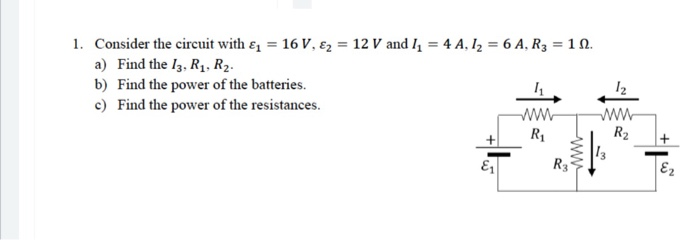# 1. Consider the circuit with &, = 16 V, €2 = 12 V and I = 4 A, Iz = 6 A, R3 = 1 N. a) Find the I3. R1. R2. b) Find the power of the batteries. c) Find the power of the resistances. R R2 R3 Ez ww

Questionhelp_outlineImage Transcriptionclose1. Consider the circuit with &, = 16 V, €2 = 12 V and I = 4 A, Iz = 6 A, R3 = 1 N. a) Find the I3. R1. R2. b) Find the power of the batteries. c) Find the power of the resistances. R R2 R3 Ez ww fullscreen

### Want to see this answer and more?

Experts are waiting 24/7 to provide step-by-step solutions in as fast as 30 minutes!*

*Response times may vary by subject and question complexity. Median response time is 34 minutes for paid subscribers and may be longer for promotional offers.
Tagged in
Engineering
Electrical Engineering

### Circuit Theory